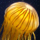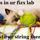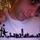## General Question# what is the square root of pi?

Asked by tunkamerica (4) November 21st, 2007
Observing members: 0Composing members: 0Are you asking for a numerical value, or for the significance of the number? Although in physics, factors of sqrt(pi) show up from time to time, there’s no deep significance to the number you get from taking the square root which is not already present for pi itself. Numerically to 500 digits, the value is:

1.77245385090551602729816748334114518279754945612238712821380778985291\
1284591032181374950656738544665416226823624282570666236152865724422602\
5250937096027870684620376986531051228499251730289508262289320953792679\
6280017463901535147972051670019018523401858544697449491264031392177552\
5906216405419332500906398407613733477475153433667989789365851836408795\
4511651617387600590673934317913328098548462481849020546548521956132515\
6164746751504273876105610799612710721006037204448367236529661370809432\
34988316684.

chris (409)“Great Answer” (0) Flag as…This is one of those questions that doesn’t have that satisfying an answer. From a purely numerical point of view, it’s just some irrational number, which means that you can’t write it as a fraction. Plug it into a calculator and you get 1.77245385091, which is a decent approximation (see chris above for a better approximation).

Geometrically, pi is the area of a circle with radius 1, so asking for the square root of pi is the same as asking for the side length of the square whose area is the same as the circle’s area. I don’t know any pressing geometric reason to do this. However, it was a famous (unsolvable) problem for many centuries to “square the circle” with a ruler and compass, which basically boils down to constructing a line segment with length sqrt(pi) exactly (or to construct the square I described above). It’s a corollary of Galois theory that this can’t be done, since pi is transcendental. Other than that, I’ve never seen a need to calculate the number specifically.

So, chris is right. There’s not too much special significance to the number that wasn’t already there in a clearer form in pi itself. Was there any reason you were curious about it?

finkelitis (1907)“Great Answer” (5) Flag as…Professors at the University of Chicago have emphasized this number as a result of an almost mystical problem (at least that is the impression they gave me).

First in an analysis course Paul Sally gave a solution to calculate the integral evaluated over -infinity to infinity of e^(-x^2) : which equaled sqrt(pi). He stressed the idea of thinking of solutions to some problems by considering auxiliary functions (in this case the gamma function as which the above problem may be rewritten).

Later in Complex Analysis, Narasimhan quoted some famous mathematician as having once said that if the above problem is not obvious to you, then you can never be a great mathematician. After that I lost most aspiration.

randomMATHfacts (5)“Great Answer” (0) Flag as…That’s bleak. The fact that sqrt(pi) pops out of the gamma function always struck me as a little mystical, though I proved it in complex analysis. It’s certainly not obvious. Maybe I’m not thinking about it right yet.

finkelitis (1907)“Great Answer” (0) Flag as…or